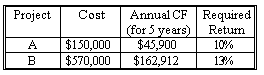### CFA Practice Question

There are 233 practice questions for this study session.

### CFA Practice Question

ABC Corp. is considering the following independent projects:Which of the following would represent the optimal choice?
A. Since Project A has an NPV of \$23,997 and Project B has an NPV of \$2,999, only Project A should be chosen.
B. Since Project A has an NPV of \$23,997 and Project B has an NPV of \$2,999, both should be chosen.
C. Since Project A has an NPV of \$23,997 and Project B has an NPV of \$47,565, both should be chosen.
Explanation: Step 1. Find NPV of each project:

Note: NPV = (PV of CF) - (Cost)

Project A: Given: PMT = 45,900; N = 5; I = 10% -> Find: PV = 173,997 -> NPV = 173,997-150,000 = 23,997

Project B: Given: PMT = 162,912; N = 5; I = 13% -> Find: PV = 572,999 -> NPV = 572,999-570,000 = 2,999

Since the projects are independent and they both have positive NPVs, the optimal choice would be to choose both.